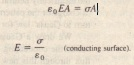# The Conductor Removed

The Conductor Removed

Suppose that by some magic the excess charges could be “frozen” into position on the conductor’s surface perhaps by embedding them in a thin ‘plastic coating and suppose that then the conductor could be removed completely This is equivalent to enlarging the cavity of until it consumes the entire conductor leaving only the charges The electric field would not change at all it would remain zero inside the thin shell of charge and would remain unchanged for all external points This shows us that the electric field is set up by the charges and not by the conductor The conductor simply provides an initial pathway for the charges to take up their position.

The External Electric Field

You have seen that the excess charge on an isolated conductor moves entirely to the conductor’s surface However unless the conductor is spherical the charge does not distribute itself uniformly Put another way the surface charge density a charge per unit area) varies over the surface of any non spherical conductor Generally this variation makes the determination of the electric field set up by the surface charges very difficult However the electric field just outside the surface of a conductor is easy to determine using Gauss’ law To do this we consider a section of the surface that is small enough to permit us to neglect any curvature and thus to take the section to be flat We then imagine a tiny cylindrical Gaussian surface to be embedded in the section as in  One end cap is fully inside the conductor the other is fully outside and the cylinder is perpendicular to the conductor’s surface  The electric field E at and just outside the conductor’s surface must also be perpendicular to that surface If it were not then it would have a component along the conductor’s surface that would exert forces on the surface charges causing them to move However such motion would violate our implicit assumption that we are dealing with electrostatic equilibrium Therefore E is perpendicular to the conductor’s surface We now sum the flux through the Gaussian surface  There is no flux through the internal end cap because the electric field within the conductor is zero There is no flux through the curved surface of the cylinder because internally in the conductor there is no electric field and externally the electric field is parallel to the curved portion of the Gaussian surface The only flux through the Gaussian surface is that through the external end cap where E is perpendicular to the plane of the cap We assume that the cap area A is small enough that the field magnitude E is constant over the cap. Then the flux through the cap is  and that is the net flux  through the Gaussian surface The charge enclosed by the Gaussian surface lies on the conductor’s surface in an area A If a is the charge per unit area then  is equal to When we substitute Gauss’ law.Perspective view (a) and side view (b) of a tiny portion of a large isolated conductor with excess positive charge on its surface A (closed) cylindrical
Gaussian surface embedded perpendicularly in the conductor encloses
some of the charge Electric field lines pierce the external end cap of the cylinder but not the internal end cap The external end cap has area A and area vector A.Thus, the magnitude of the electric field at a location just outside a conductor is proportional to the surface charge density at that location on the conductor If the charge on the conductor is positive, the electric field is directed away from the conductor as in It is directed toward the conductor if the charge is negative The field line in must terminate on negative charges somewhere in the environment If we bring hose charges near the conductor the charge density at any given location on the conductor surface changes and so does the magnitude of the electric field. However the relation between a and E is still given.